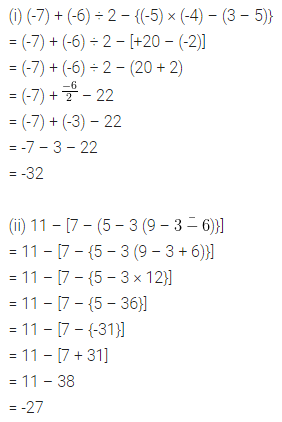# ML Aggarwal Class 7 Solutions for ICSE Maths Chapter 1 Integers Check Your Progress

## ML Aggarwal Class 7 Solutions for ICSE Maths Chapter 1 Integers Check Your Progress

Question 1.
Evaluate the following:
(i) (-7) × (-9) × (-11)
(ii) (-5) × 7 × (-6) × (-8)
(iii) (-1024) ÷ 32
(iv) (-216) ÷ (-12)
Solution: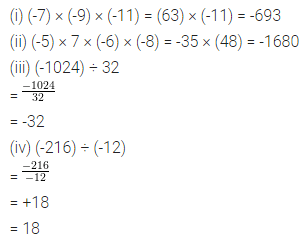Question 2.
What will be the sign of the product if we multiply 39 negative integers and 98 positive integers?
Solution: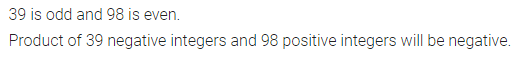Question 3.
Use the sign >, < or = in the box to make the following statements true:
(i) (-15) + 38 ……… 27 + (-50)
(ii) (-13) × 0 × (-5) …….. (-7) × (-6) × 14
(iii) (-18) ÷ (-3) …….. (-10) + (-15) + 31
(iv) (-5) × (-7) × (-10) …….. (-1400) ÷ (-4)
Solution: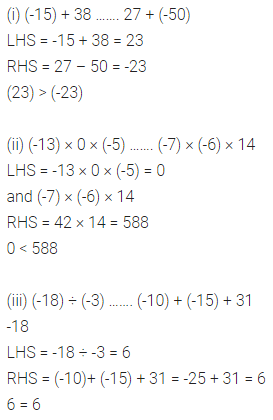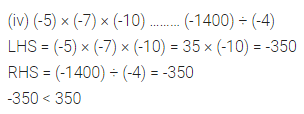Question 4.
Is {(-45) ÷ (-15)} ÷ (-3) = (-45) ÷ [(-15) ÷ (-3)]?
Solution: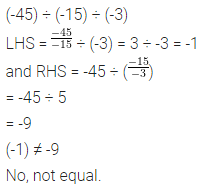Question 5.
A cement company earns a profit of ₹ 8 per bag of white cement sold and a loss of ₹ 5 per bag of grey cement sold.
(i) The company sells 3000 bags of white cement and 5000 bags of grey cement in a month. What is its profit or loss?
(ii) What is the number of white cement bags it must sell to have neither profit nor loss if the number of grey cement bags sold is 6400?
Solution: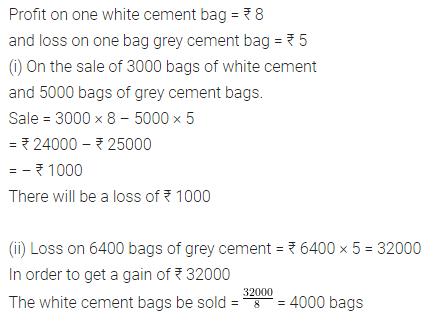Question 6.
Simplify the following:
(i) (-7) + (-6) ÷ 2 – {(-5) × (-4) – (3 – 5)}
(ii) 11 – [7 – (5 – 3 (9 – $$\bar { 3-6 }$$)}].
Solution: